# Electric Forces and Electric Fields Objective Define and

• Slides: 43Electric Forces and Electric Fields Objective: • Define and apply Coulomb’s Law to calculate the electric force between charged particles. • Plot electric field lines and calculate electric fields for simple charge distributions.Bellwork – please write the answer on your homework 1. A glass rod rubbed with silk acquires a charge of +8. 0 x 10 -10 C. a) What is the charge on the silk and how many electrons have been transferred to the silk? b) How much mass has the glass rod lost? 2. Fiona is on her way home but must leave her new-found love, Shrek, behind. As Fiona’s stallion takes off at 4 m/s, Shrek tosses a bouquet of onion blossoms with a speed of 6 m/s. According to Fiona, how fast are the flowers when she catches them? 3. In an attempt to separate the two, Fairy Godmother casts a spell that speeds up the stallion to 70% the speed of light. It takes 20 hours, according to Fiona’s watch (t 0 ) to break the spell. How long did it take according to Shrek’s clock?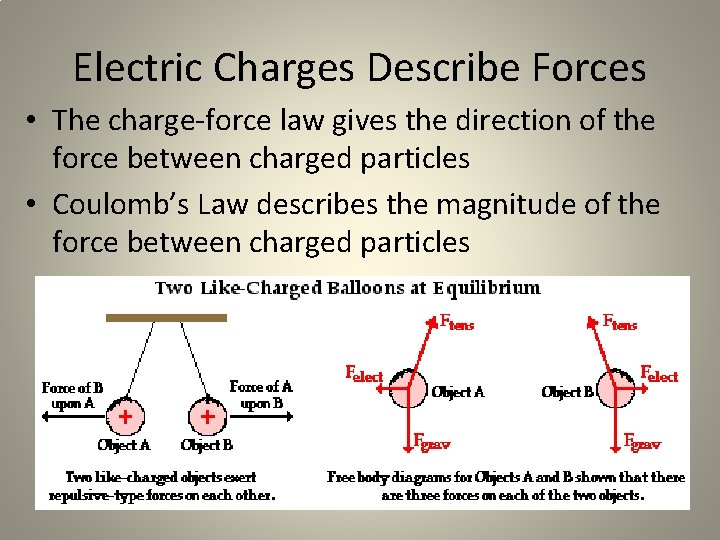Electric Charges Describe Forces • The charge-force law gives the direction of the force between charged particles • Coulomb’s Law describes the magnitude of the force between charged particlesCoulomb’s Law • The magnitude of electrical force between two point charges is directly proportional to the product of the magnitude of the charges themselves and inversely proportional to the square of the distance between them. • k is a constant whose experimental value was determined by Coulomb to be k=8. 988 x 109 Nm 2/C 2 .Sketching Free-Body Diagrams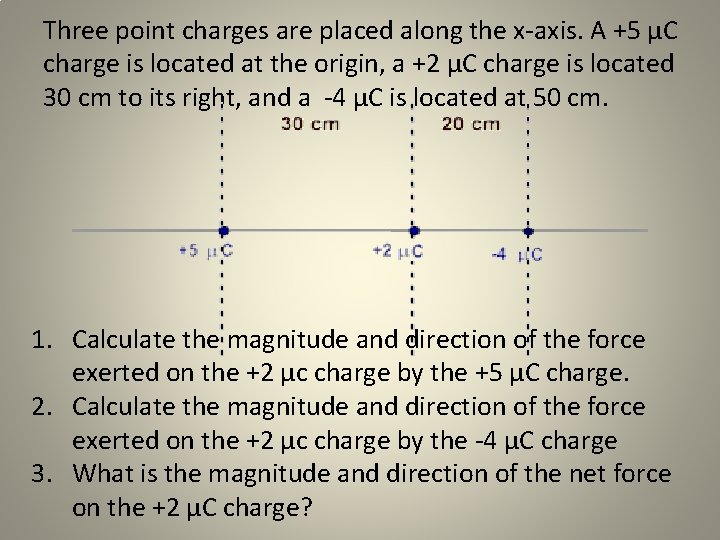Three point charges are placed along the x-axis. A +5 µC charge is located at the origin, a +2 µC charge is located 30 cm to its right, and a -4 µC is located at 50 cm. 1. Calculate the magnitude and direction of the force exerted on the +2 µc charge by the +5 µC charge. 2. Calculate the magnitude and direction of the force exerted on the +2 µc charge by the -4 µC charge 3. What is the magnitude and direction of the net force on the +2 µC charge?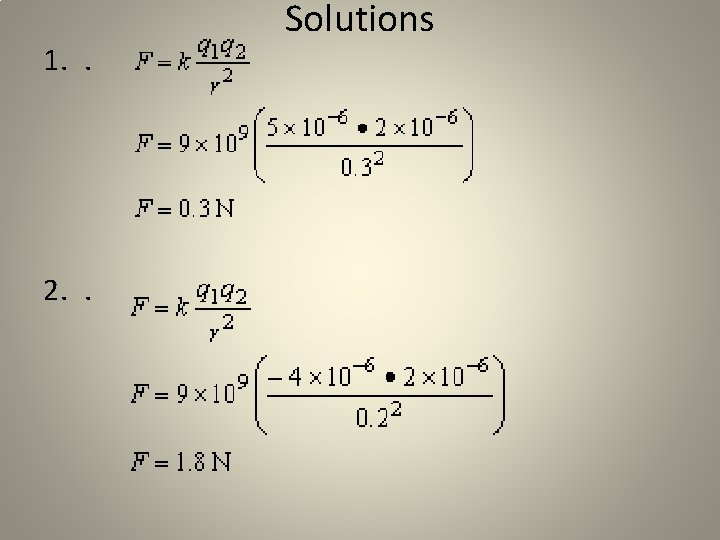1. . 2. . Solutions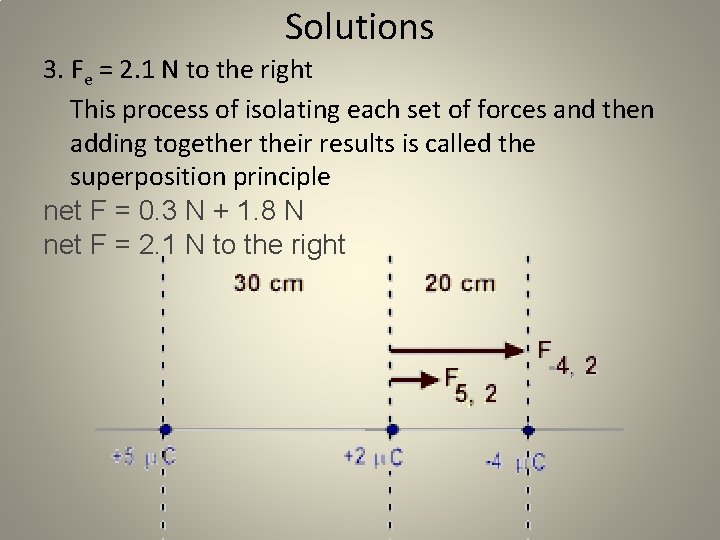Solutions 3. Fe = 2. 1 N to the right This process of isolating each set of forces and then adding together their results is called the superposition principle net F = 0. 3 N + 1. 8 N net F = 2. 1 N to the right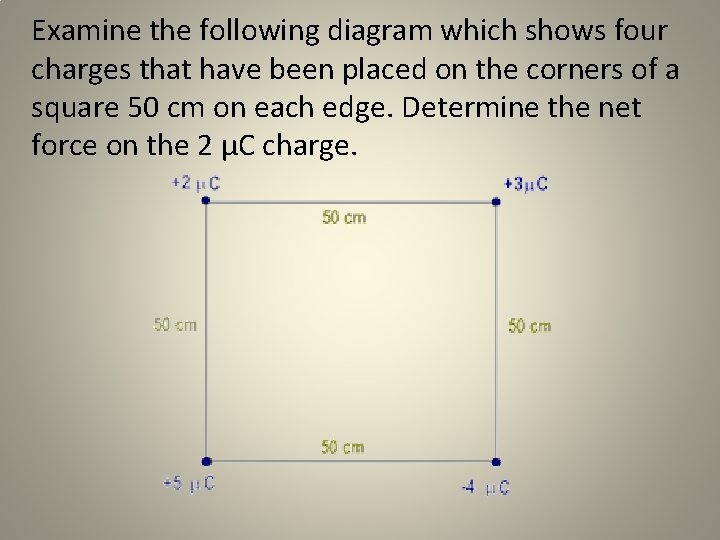Examine the following diagram which shows four charges that have been placed on the corners of a square 50 cm on each edge. Determine the net force on the 2 µC charge.Problem-Solving Strategy 1. Sketch the free-body diagram to describe the forces on the 2 μC charge 2. Calculate the magnitude and direction of F 3, 2 3. Calculate the magnitude and direction of F 5, 2 4. Calculate the magnitude and direction of F-4, 2 5. Calculate the net force on the 2 µC charge in both the x-direction and the y-direction 6. Calculate the final magnitude and direction of the force on the 2 µC charge.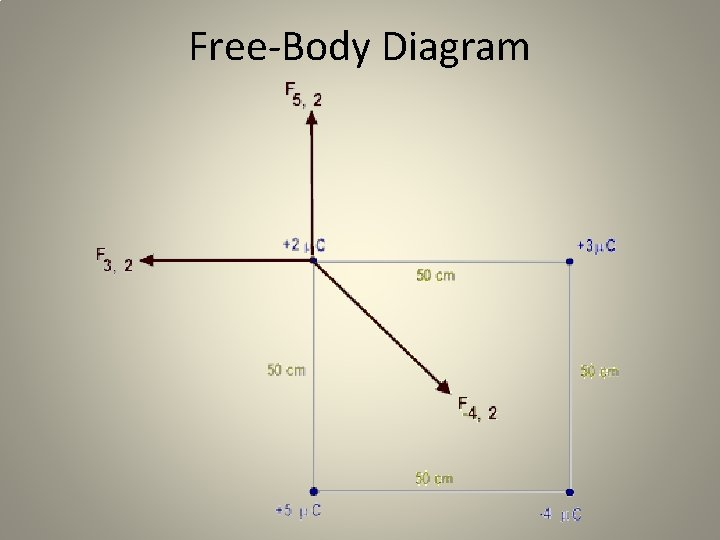Free-Body Diagram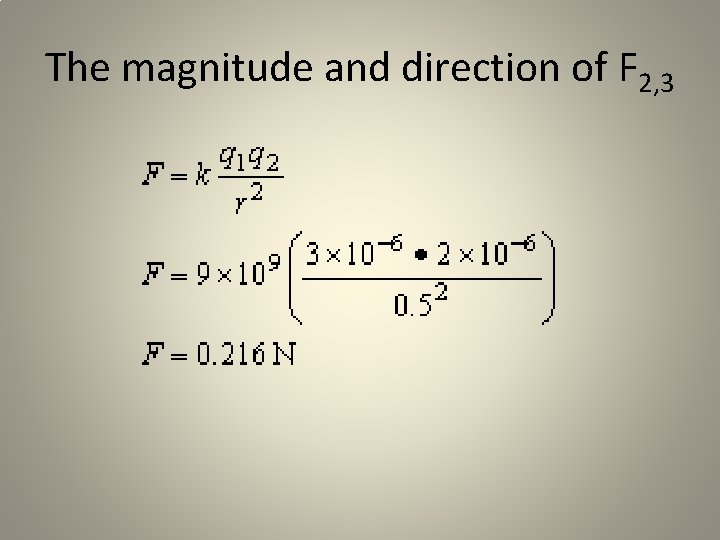The magnitude and direction of F 2, 3The magnitude and direction of F 5, 2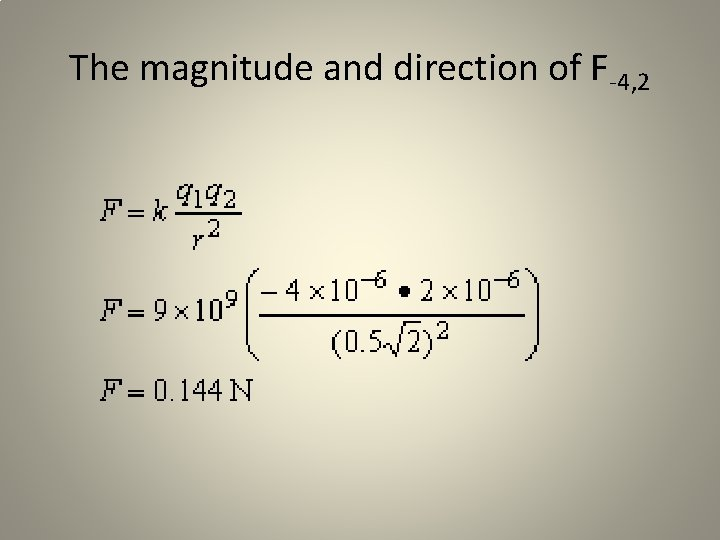The magnitude and direction of F-4, 2Evaluate components of the net force F x y F 5, 2 0 0. 36 F 3, 2 -0. 216 0 F-4, 2 0. 144 cos 45º = 0. 102 -0. 144 sin 45º = -0. 102 Fnet -0. 114 N 0. 258 N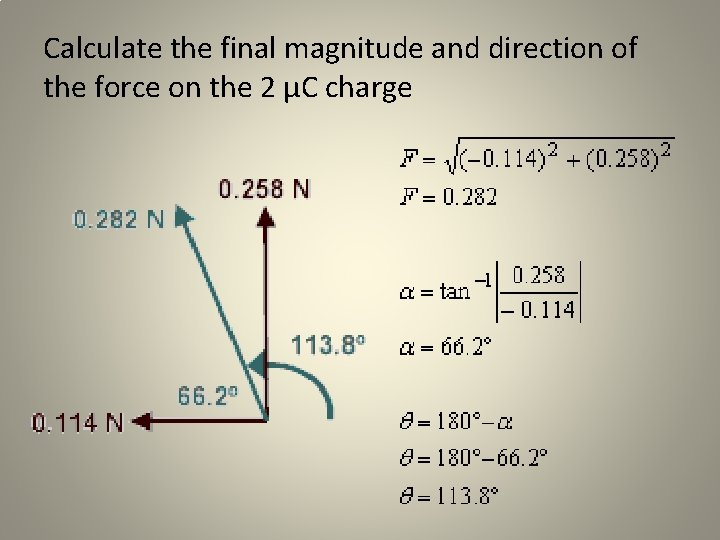Calculate the final magnitude and direction of the force on the 2 µC chargeElectric Fields • An electric field is the region surrounding a charged particle, Q, where another charged particle with experience either a force of attraction or repulsion • The magnitude of the electric field strength, E, is E= k. Q/r 2 • The SI Units are derived by: (Nm 2/C 2)(C)(1/m 2) = N/C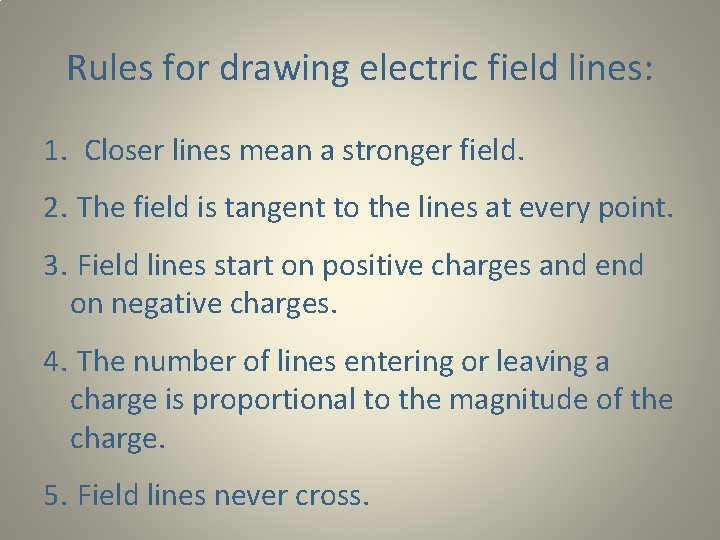Rules for drawing electric field lines: 1. Closer lines mean a stronger field. 2. The field is tangent to the lines at every point. 3. Field lines start on positive charges and end on negative charges. 4. The number of lines entering or leaving a charge is proportional to the magnitude of the charge. 5. Field lines never cross.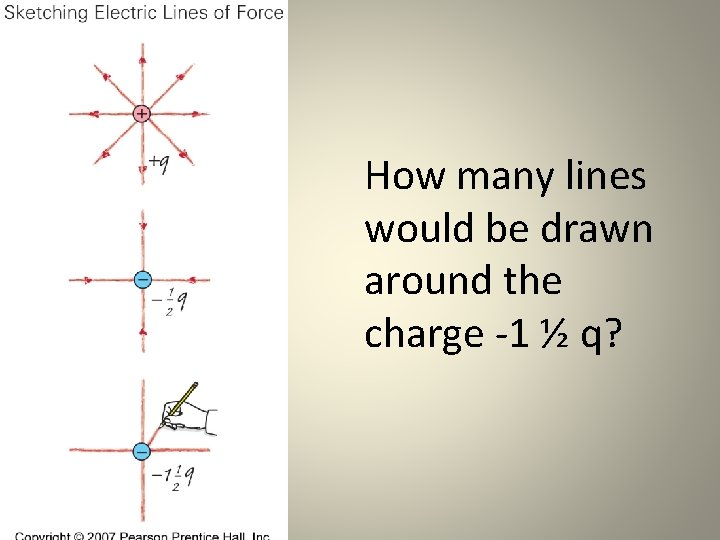How many lines would be drawn around the charge -1 ½ q?Electric Field Lines for Configurations of Two or More Charges oppositely-charged particles like-charged particles Left is +, right is both are + • Fields between oppositely charged particles are attractive and are elliptical in shape; while fields between similarly charged particles are repulsive and hyperbolic in shape.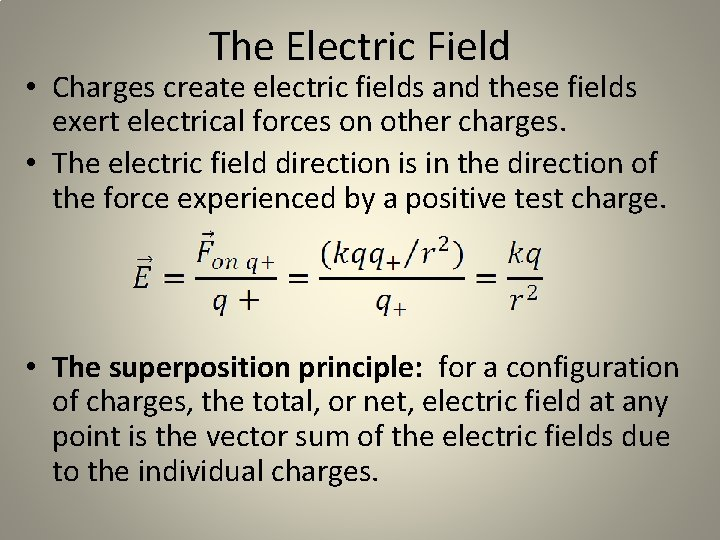The Electric Field • Charges create electric fields and these fields exert electrical forces on other charges. • The electric field direction is in the direction of the force experienced by a positive test charge. • The superposition principle: for a configuration of charges, the total, or net, electric field at any point is the vector sum of the electric fields due to the individual charges.Bellwork and Quiz Review In a 4. 00 g of helium, the nuclei are separated from the electrons by a distance of 1 km. What is the magnitude of the electric force between the protons and the electrons?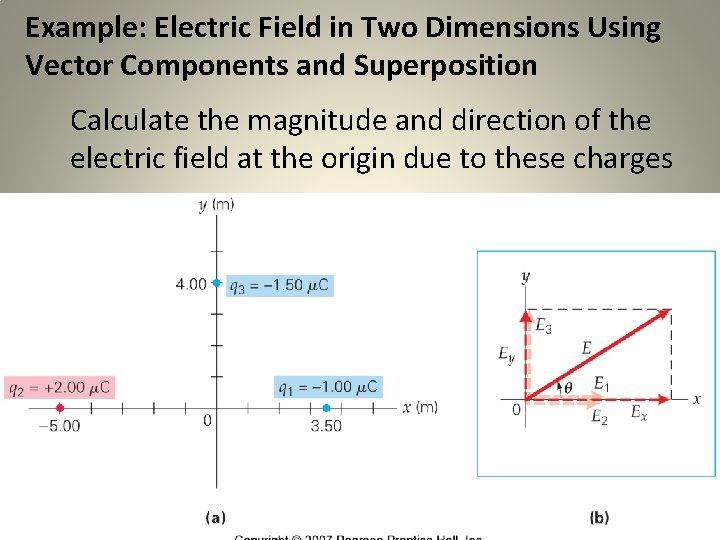Example: Electric Field in Two Dimensions Using Vector Components and Superposition Calculate the magnitude and direction of the electric field at the origin due to these charges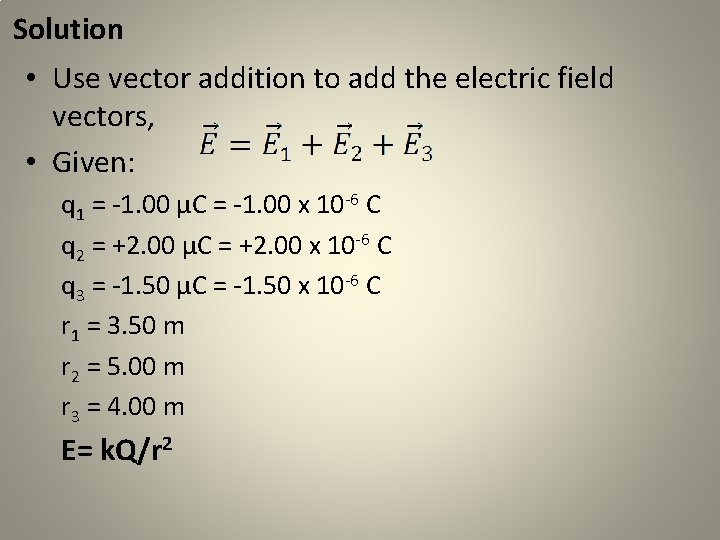Solution • Use vector addition to add the electric field vectors, • Given: q 1 = -1. 00 μC = -1. 00 x 10 -6 C q 2 = +2. 00 μC = +2. 00 x 10 -6 C q 3 = -1. 50 μC = -1. 50 x 10 -6 C r 1 = 3. 50 m r 2 = 5. 00 m r 3 = 4. 00 m E= k. Q/r 2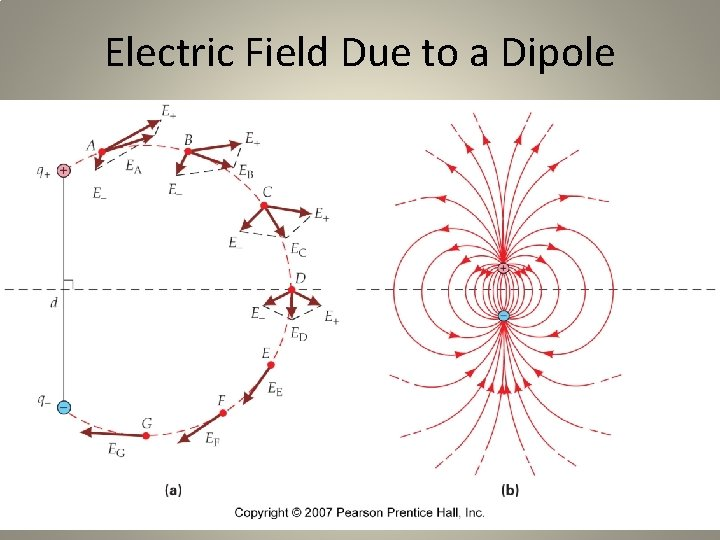Electric Field Due to a DipoleElectric Field Due to Parallel Plates: when two parallel plates are connected across a battery, the plates become charged an electric field is established between them. • the direction of an electric field is defined as the direction that a positive test charge would move. • The electric field would point from the positive plate to the negative plate. • Since the field lines are parallel to each other, this type of electric field is uniform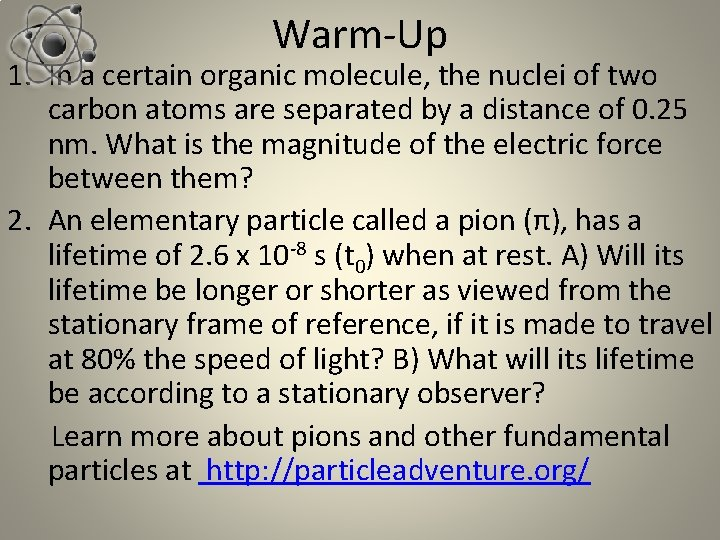Warm-Up 1. In a certain organic molecule, the nuclei of two carbon atoms are separated by a distance of 0. 25 nm. What is the magnitude of the electric force between them? 2. An elementary particle called a pion (π), has a lifetime of 2. 6 x 10 -8 s (t 0) when at rest. A) Will its lifetime be longer or shorter as viewed from the stationary frame of reference, if it is made to travel at 80% the speed of light? B) What will its lifetime be according to a stationary observer? Learn more about pions and other fundamental particles at http: //particleadventure. org/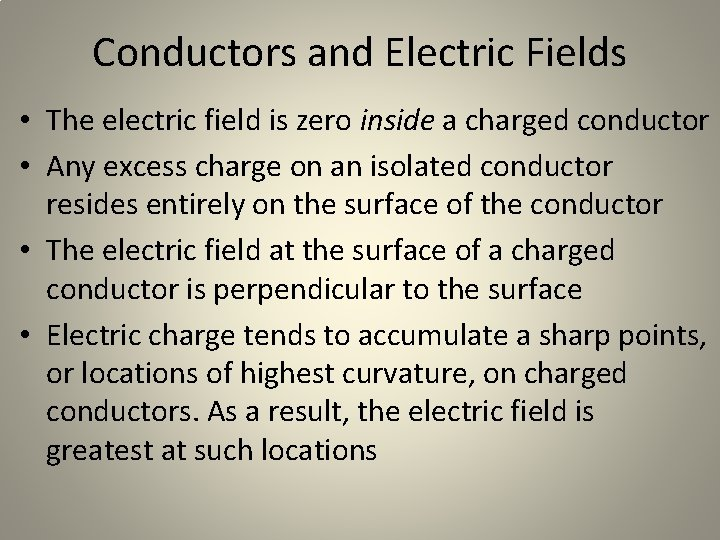Conductors and Electric Fields • The electric field is zero inside a charged conductor • Any excess charge on an isolated conductor resides entirely on the surface of the conductor • The electric field at the surface of a charged conductor is perpendicular to the surface • Electric charge tends to accumulate a sharp points, or locations of highest curvature, on charged conductors. As a result, the electric field is greatest at such locations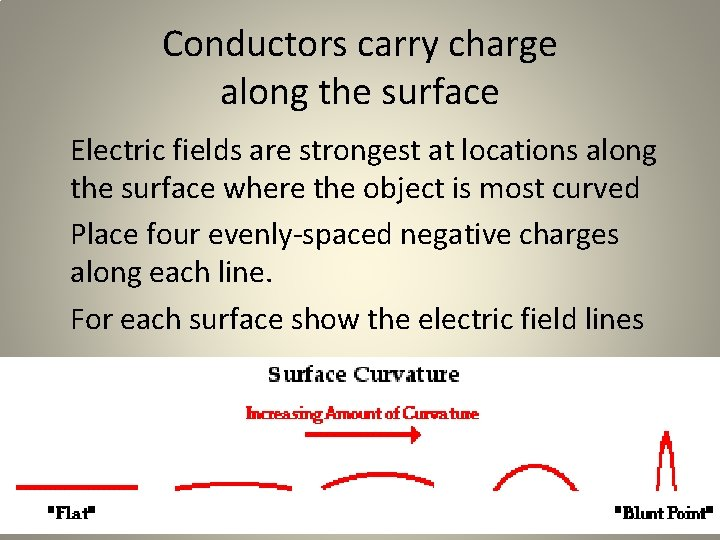Conductors carry charge along the surface Electric fields are strongest at locations along the surface where the object is most curved Place four evenly-spaced negative charges along each line. For each surface show the electric field lines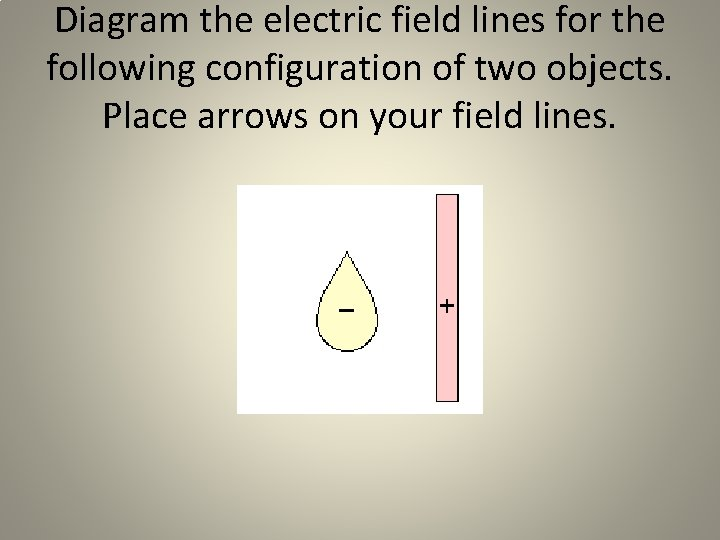Diagram the electric field lines for the following configuration of two objects. Place arrows on your field lines.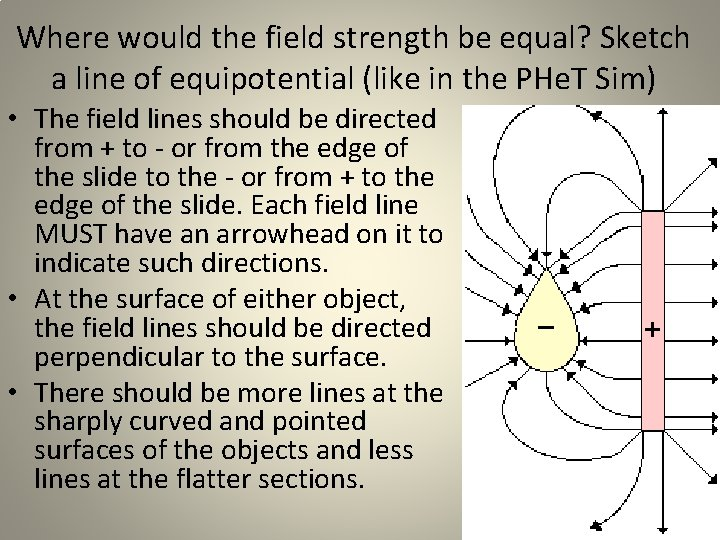Where would the field strength be equal? Sketch a line of equipotential (like in the PHe. T Sim) • The field lines should be directed from + to - or from the edge of the slide to the - or from + to the edge of the slide. Each field line MUST have an arrowhead on it to indicate such directions. • At the surface of either object, the field lines should be directed perpendicular to the surface. • There should be more lines at the sharply curved and pointed surfaces of the objects and less lines at the flatter sections.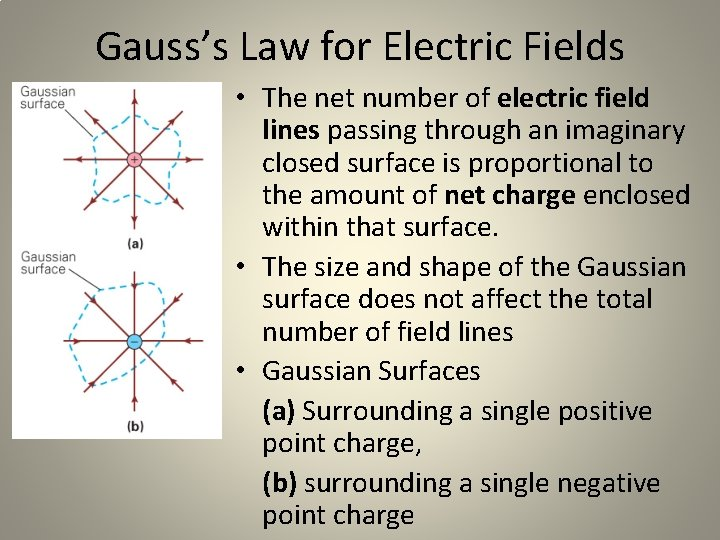Gauss’s Law for Electric Fields • The net number of electric field lines passing through an imaginary closed surface is proportional to the amount of net charge enclosed within that surface. • The size and shape of the Gaussian surface does not affect the total number of field lines • Gaussian Surfaces (a) Surrounding a single positive point charge, (b) surrounding a single negative point charge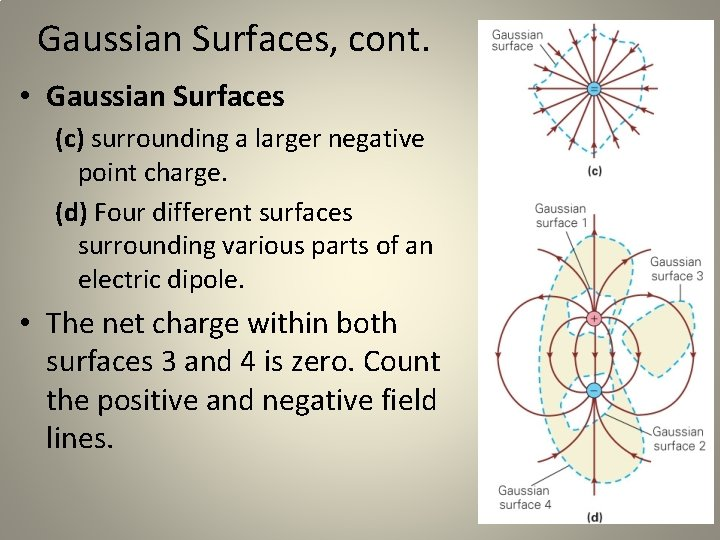Gaussian Surfaces, cont. • Gaussian Surfaces (c) surrounding a larger negative point charge. (d) Four different surfaces surrounding various parts of an electric dipole. • The net charge within both surfaces 3 and 4 is zero. Count the positive and negative field lines.Conservation of Energy • It would take work to move two like charges closer together or to separate opposite charges • How much work? Work = some force applied across a given distance • W = Fcosqd • Energy is the ability to do work. • W = DKE = KEfinal - KEinitial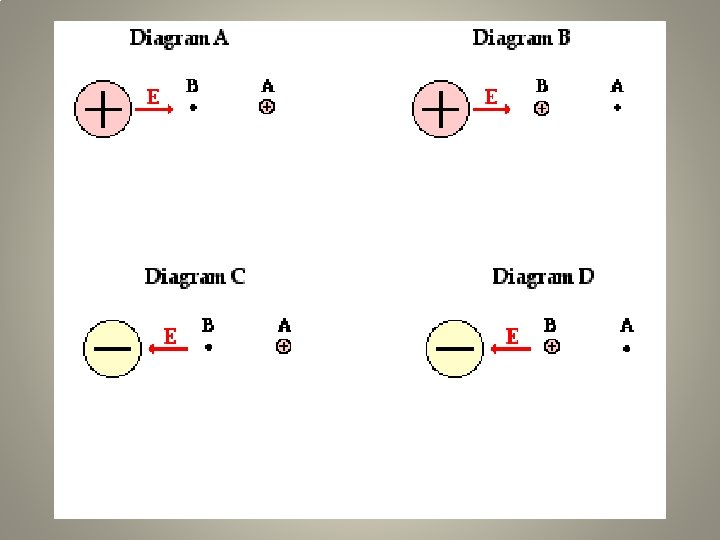Electric Potential The Potential Energy (Work) to move charges through an electrical field depends on: 1) Electric charge - a property of the object experiencing the electrical field 2) Distance from source - the location within the electric field and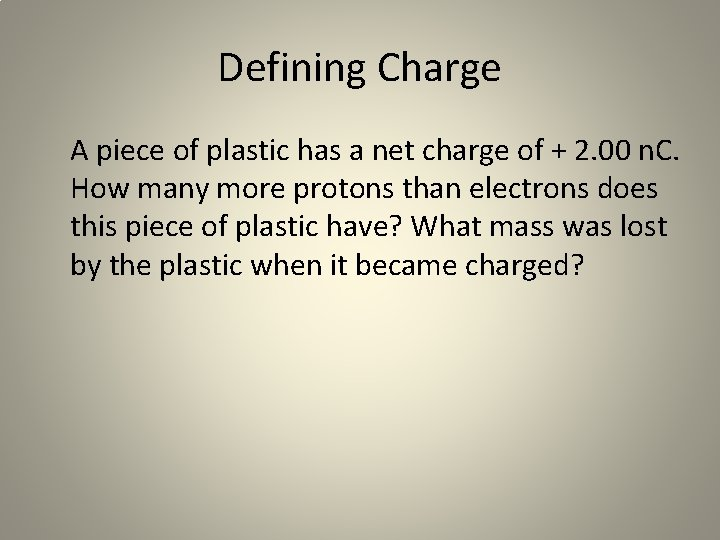Defining Charge A piece of plastic has a net charge of + 2. 00 n. C. How many more protons than electrons does this piece of plastic have? What mass was lost by the plastic when it became charged?Electrical Forces: Applications of Coulomb’s Law Two 1. 2 -gram balloons are suspended from light strings attached to the ceiling at the same point. The net charge on the balloons is -540 n. C. The balloons are distanced 68. 2 cm apart when at equilibrium. Determine the amount of tension on the string.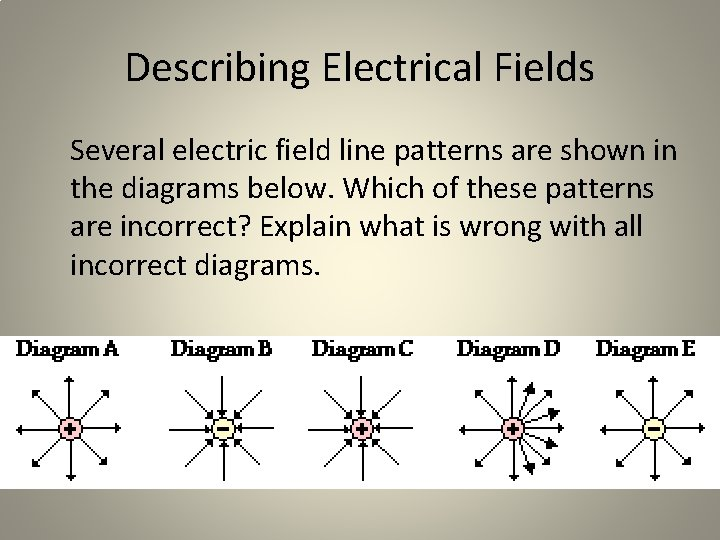Describing Electrical Fields Several electric field line patterns are shown in the diagrams below. Which of these patterns are incorrect? Explain what is wrong with all incorrect diagrams.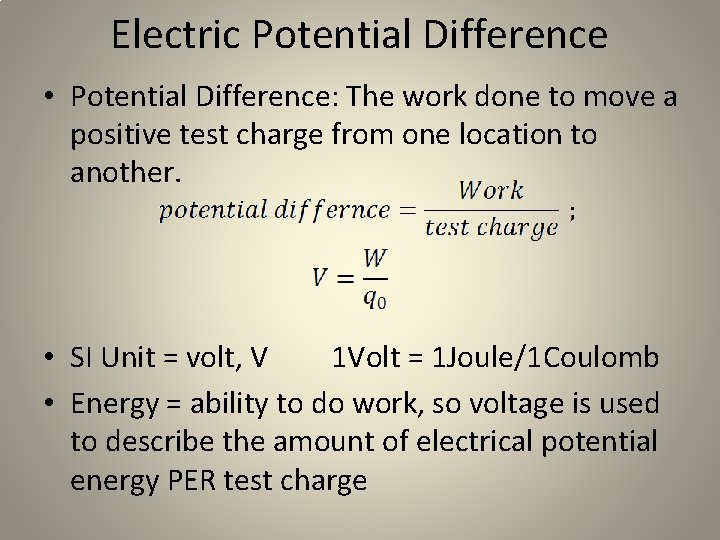Electric Potential Difference • Potential Difference: The work done to move a positive test charge from one location to another. • SI Unit = volt, V 1 Volt = 1 Joule/1 Coulomb • Energy = ability to do work, so voltage is used to describe the amount of electrical potential energy PER test charge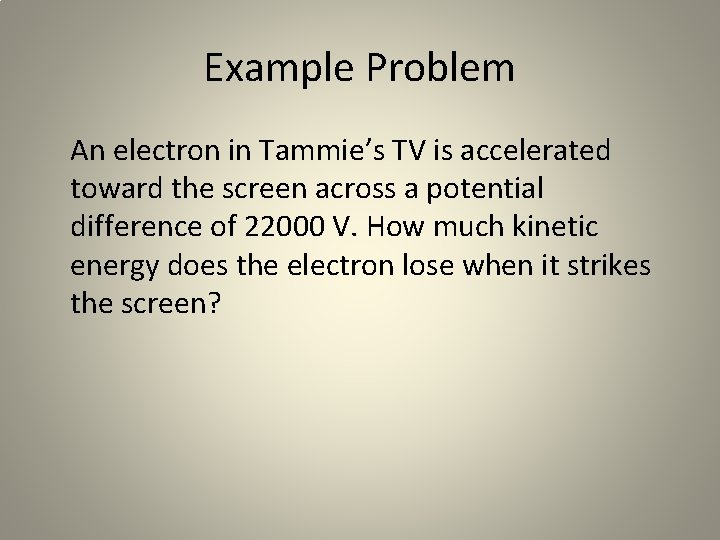Example Problem An electron in Tammie’s TV is accelerated toward the screen across a potential difference of 22000 V. How much kinetic energy does the electron lose when it strikes the screen?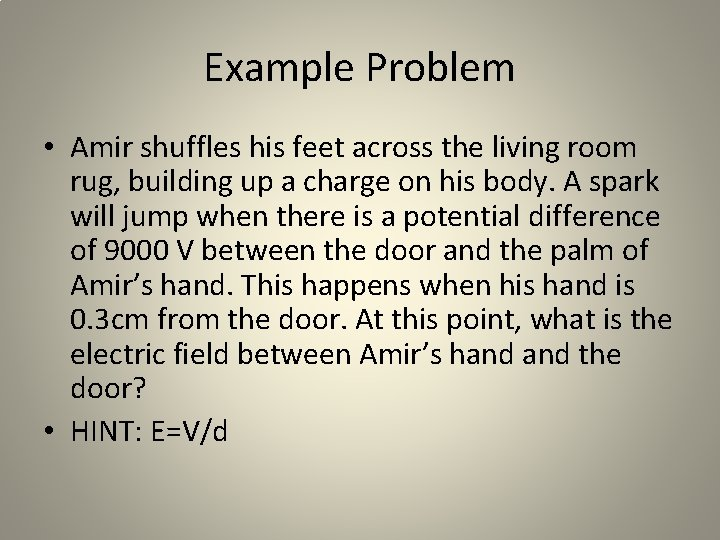Example Problem • Amir shuffles his feet across the living room rug, building up a charge on his body. A spark will jump when there is a potential difference of 9000 V between the door and the palm of Amir’s hand. This happens when his hand is 0. 3 cm from the door. At this point, what is the electric field between Amir’s hand the door? • HINT: E=V/dIndependent Practice p. 531 – 535; 28, 29, 40, 52, 70, 77, 85 Finish “Electric Fields – Point Charges” Review for Ch 15 Quiz Thursday Set up your lab notebook to collect Egg Car Data and update your journaling section. This is worth 20 points, so be thorough and include relevant details in your observations and notes! • Be ready for Egg Car Collisions Test Runs Friday! • •